Question

# If 76g of ice at 0.0oC is placed in 245mL of water at 35oC, find the...

If 76g of ice at 0.0oC is placed in 245mL of water at 35oC, find the final temperature if all of the ice melts. All the necessary constants are in your textbook.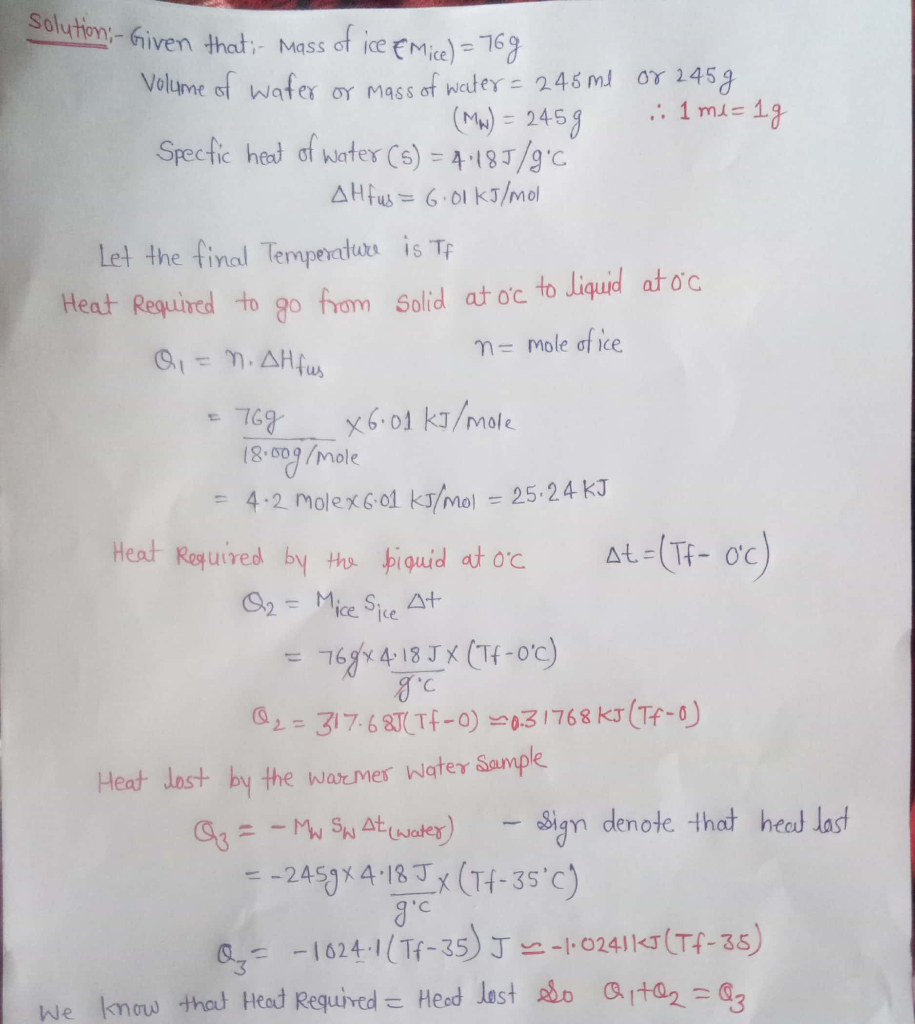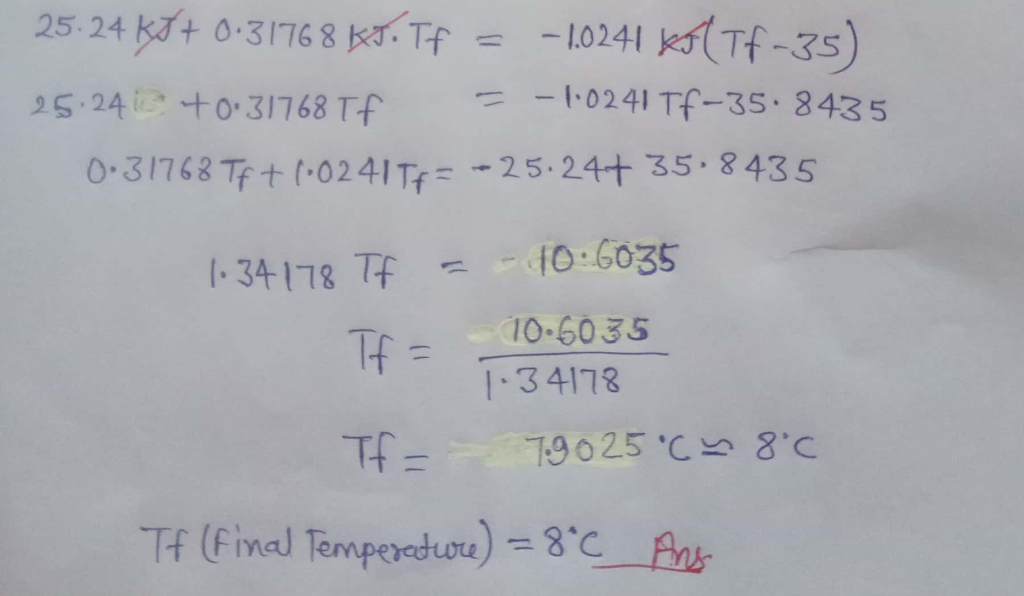#### Earn Coins

Coins can be redeemed for fabulous gifts.

Similar Homework Help Questions
• ### Two 20.0-g ice cubes at –13.0 °C are placed into 225 g of water at 25.0...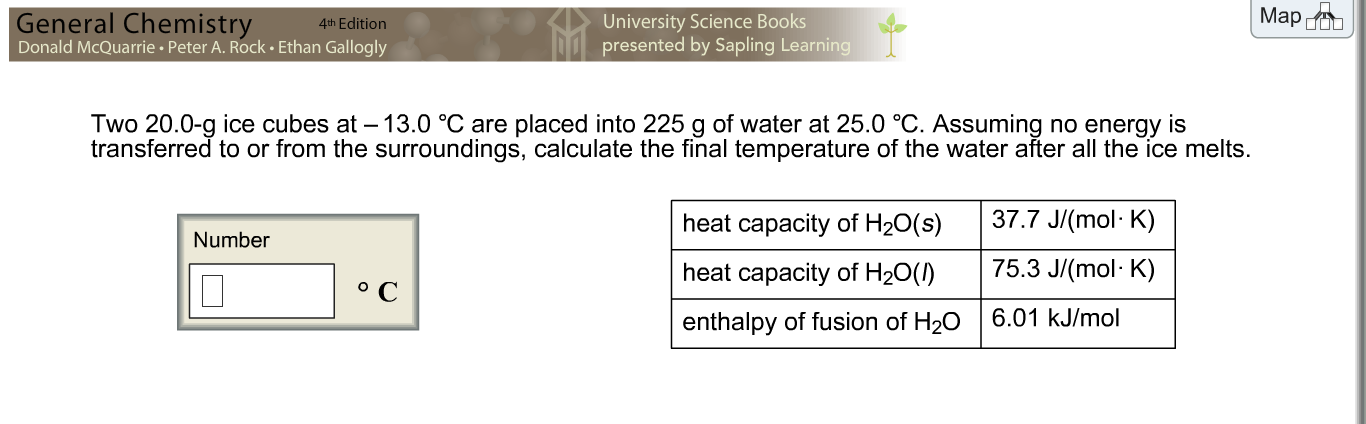Two 20.0-g ice cubes at –13.0 °C are placed into 225 g of water at 25.0 °C. Assuming no energy is transferred to or from the surroundings, calculate the final temperature of the water after all the ice melts. PLEASE SHOW WORK!

• ### if 65g of ice at 0.0 degrees celsius is placed in 245mL of water at 35...

if 65g of ice at 0.0 degrees celsius is placed in 245mL of water at 35 degrees celsius, find the final temperature if all of the ice melts. STEP BY STEP PLEASE

• ### Two 20.0 g ice cubes at −18.0 ∘C are placed into 275 g of water at...

Two 20.0 g ice cubes at −18.0 ∘C are placed into 275 g of water at 25.0 ∘C. Assuming no energy is transferred to or from the surroundings, calculate the final temperature, ?f, of the water after all the ice melts.

• ### Two 20.0-g ice cubes at –16.0 °C are placed into 265 g of water at 25.0...

Two 20.0-g ice cubes at –16.0 °C are placed into 265 g of water at 25.0 °C. Assuming no energy is transferred to or from the surroundings, calculate the final temperature of the water after all the ice melts.

• ### Two 20.0-g ice cubes at -10.0 degree C are placed into 205 g of water at...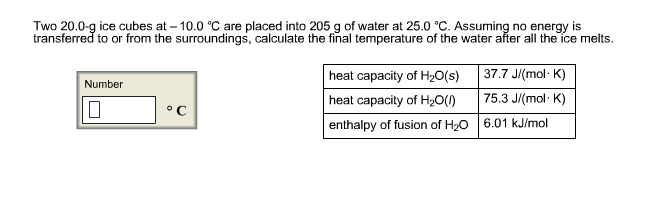Two 20.0-g ice cubes at -10.0 degree C are placed into 205 g of water at 25.0 degree C. Assuming no energy is transferred to or from the surroundings, calculate the final temperature of the water after all the ice melts.

• ### Two 20.0-g ice cubes at -14.0 degree C are placed into 295 g of water at...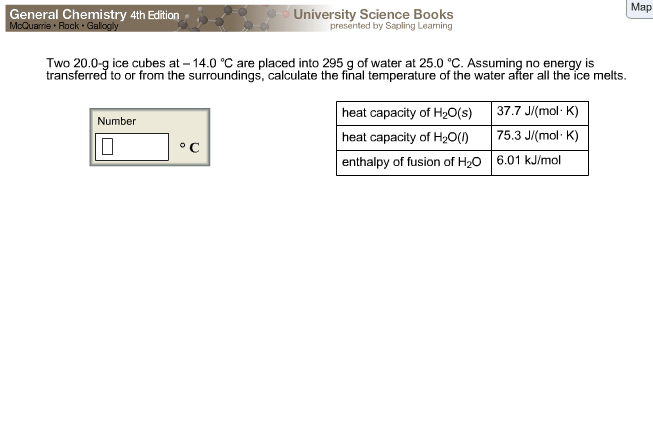Two 20.0-g ice cubes at -14.0 degree C are placed into 295 g of water at 25.0 degree C. Assuming no energy is transferred to or from the surroundings, calculate the final temperature of the water after all the ice melts.

• ### Two 20.0-g ice cubes at –10.0 °C are placed into 255 g of water at 25.0...

Two 20.0-g ice cubes at –10.0 °C are placed into 255 g of water at 25.0 °C. Assuming no energy is transferred to or from the surroundings, calculate the final temperature of the water after all the ice melts. heat capacity of water s = 37.7 heat capacity of water q =75.3 fusion = 6.01

• ### by Two 20 0g ice cubes at-13.0 ℃ are placed into 205 g of water at...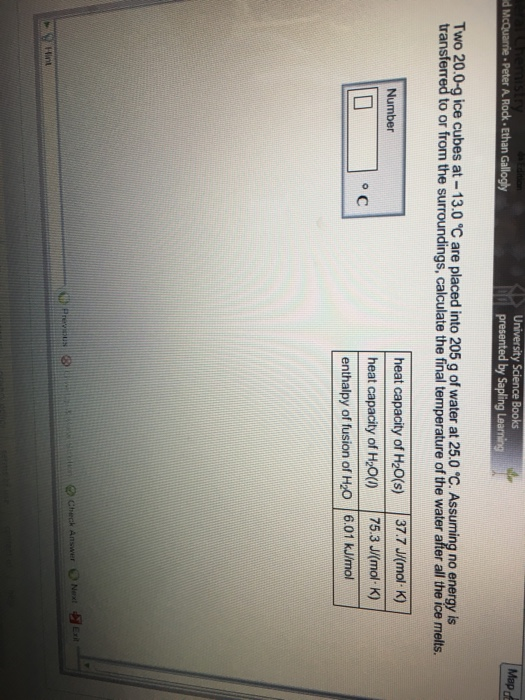by Two 20 0g ice cubes at-13.0 ℃ are placed into 205 g of water at 25.0 ℃ Assuming no transferred to or from the surroundings, calculate the final temperature of the water after all the ice melts heat capacity of H20(s) 37.7J(mol K) heat capacity of H20() 75.3 J(mol K) enthalpy of fusion of H2O 6.01 kJ/mol

• ### A 210 g piece of ice at 0°C is placed in 480 g of water at...

A 210 g piece of ice at 0°C is placed in 480 g of water at 25°C. The system is in a container of negligible heat capacity and is insulated from its surroundings. (a) What is the final equilibrium temperature of the system? °C (b) How much of the ice melts? g

• ### Two 20.0 g ice cubes at -12.0 °C are placed into 225 g of water at...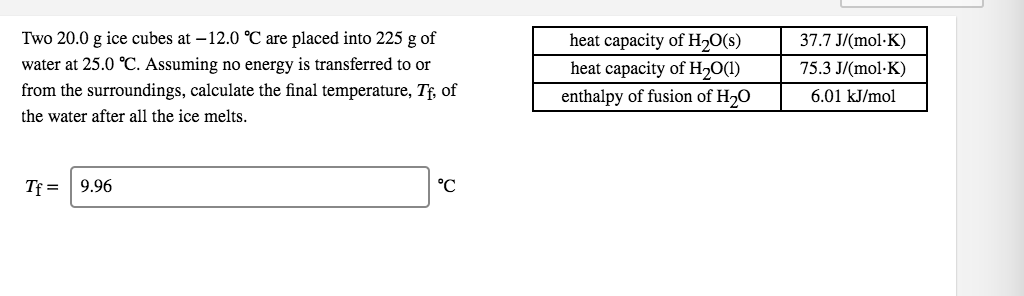Two 20.0 g ice cubes at -12.0 °C are placed into 225 g of water at 25.0 °C. Assuming no energy is transferred to or from the surroundings, calculate the final temperature, Tf, of the water after all the ice melts. heat capacity of H2O(s) heat capacity of H2O(1) enthalpy of fusion of H20 37.7 J/(molK) 75.3 J/(mol·K) 6.01 kJ/mol Tf= 9.96